﻿ 一个二维对流扩散方程源项反问题的条件唯一性 Conditional Uniqueness for an Inverse Source Problem in the Two Dimensional Convection-Diffusion Equations

Vol.05 No.04(2016), Article ID:18925,7 pages
10.12677/AAM.2016.54068

Conditional Uniqueness for an Inverse Source Problem in the Two Dimensional Convection-Diffusion Equations

Qian Liu, Zhendong Wang, Gongsheng Li*

School of Sciences, Shandong University of Technology, Zibo Shandong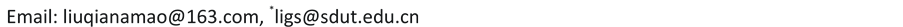Received: Oct. 19th, 2016; accepted: Nov. 7th, 2016; published: Nov. 11th, 2016ABSTRACT

This article deals with an inverse problem of determining a continuous linear source term in the two dimensional convection-diffusion equation by using the variational adjoint method. A variational identity connecting the known data with the unknown is established based on an adjoint problem, and the conditional uniqueness to the inverse source problem is proved by the approximate controllability to the adjoint problem under the condition that the inverse solution can keep orders locally.

Keywords:Convection-Diffusion Equation, Inverse Source Problem, Adjoint Problem, Variational Identity, Conditional Uniqueness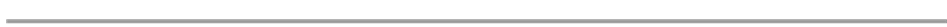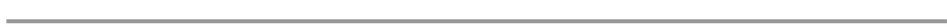1. 引言

2. 正问题与反问题

2.1. 正问题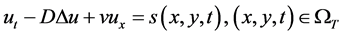， (2.1)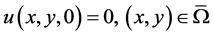， (2.2)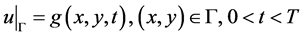。 (2.3)

2.2. 反问题， (2.4)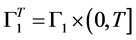。设为源项强度函数的容许集，其中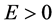为一个正常数。则对于任意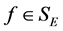，正问题有唯一解，记为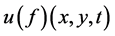。联系到附加数据条件，定义映射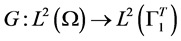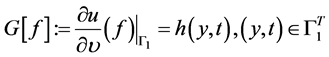。(2.5)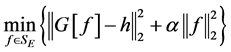，(2.6)(2.7)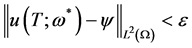3. 反问题解的唯一性

3.1. 变分恒等式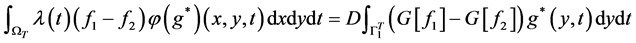， (3.1)(3.2)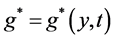为可控制的边界输入函数。(3.3)。 (3.4)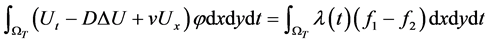。 (3.5)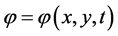是伴随问题(3.2)的解，则联系到条件(3.4)式，由(3.5)式即得(3.1)式。由于伴随问题(3.2) 的解由边值函数所确定，其解记为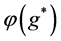。定理证毕。

3.2. 条件唯一性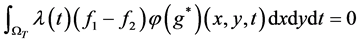。 (3.6)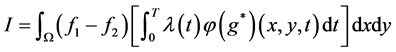。 (3.7)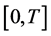的一个剖分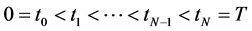， (3.8)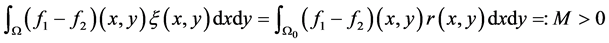。 (3.9)。 (3.10)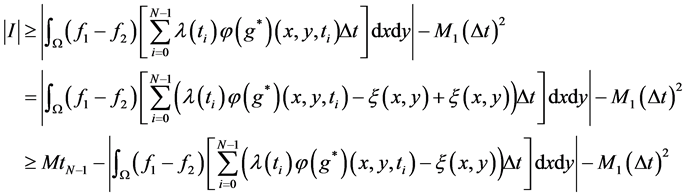。 (3.11)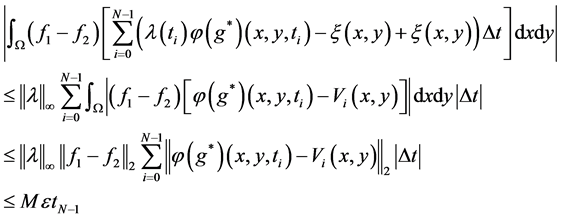。 (3.12)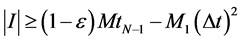。 (3.13)

4. 结论

Conditional Uniqueness for an Inverse Source Problem in the Two Dimensional Convection-Diffusion Equations[J]. 应用数学进展, 2016, 05(04): 591-597. http://dx.doi.org/10.12677/AAM.2016.54068

1. 1. Isakov, V. (1998) Inverse Problems for Partial Differential Equations. Spring-Verlag, New York. http://dx.doi.org/10.1007/978-1-4899-0030-2

2. 2. 李功胜, 姚德. 扩散模型的源项反演及其应用[M]. 北京: 科学出版社, 2014.

3. 3. Isakov, V. (1991) Inverse Parabolic Problems with the Final Overdetermination. Communications on Pure and Applied Mathematics, 54, 185-209.

4. 4. Yamamoto, M. (1993) Conditional Stability in Determination of Force Terms of Heat Equations in a Rectangle. Mathematical and Computer Modelling, 18, 79-88.

5. 5. Isakov, V. (1995) Global Uniqueness for Inverse Parabolic Problems with Final Observation. Inverse Problems, 11, L11-L16. http://dx.doi.org/10.1088/0266-5611/11/4/001

6. 6. Isakov, V. (1999) Some Inverse Problems for the Diffusion Equation. Inverse Problems, 15, 3-10. http://dx.doi.org/10.1088/0266-5611/15/1/004

7. 7. Choulli, M. and Yamamoto, M. (2004) Conditional Stability in Determining a Heat Source. Journal of Inverse and Ill-Posed Problems, 12, 233-244. http://dx.doi.org/10.1515/1569394042215856

8. 8. DuChateau, P. and Rundell, W. (1985) Unicity in an Inverse Problem for an Unknown Term in a Reaction Diffusion Equation. Journal of Differential Equations, 59, 155-164. http://dx.doi.org/10.1016/0022-0396(85)90152-4

9. 9. DuChateau, P. (1995) Monotomicity and invertibility of Coefficient to Data Mapping for Parabolic Inverse Problems. SIAM Journal on Mathematical Analysis, 26, 1473-1487. http://dx.doi.org/10.1137/S0036141093259257

10. 10. DuChateau, P. (1997) An Inverse Problem for the Hydraulic Properties of Porous Media. SIAM Journal on Mathematical Analysis, 28, 611-632. http://dx.doi.org/10.1137/S0036141095285673

11. 11. DuChateau, P., Thelwell, R. and Butters, M. (2004) Analysis of an Adjoint Problem Approach to the Identification of an Unknown Diffusion Coefficient. Inverse Problems, 20, 601-625. http://dx.doi.org/10.1088/0266-5611/20/2/019

12. 12. Hasanov, A., DuChateau, P. and Petkas, B. (2006) An Adjoint Problem Approach and Coarsefine Mesh Method for Identification of the Diffusion in a Linear Parabolic Equation. Journal of Inverse and Ill-Posed Problems, 14, 435-463. http://dx.doi.org/10.1515/156939406778247615

13. 13. Li, G.S. and Yamamoto, M. (2006) Stability Analysis for Determining a Source Term in a 1-D Advection-Dispersion Equation. Journal of Inverse and Ill-Posed Problems, 14, 147-155.http://dx.doi.org/10.1515/156939406777571067

14. 14. DuChateau, P. (2013) An Adjoint Method for Proving Identifiability of Coefficients in Parabolic Equations. Journal of Inverse and Ill-Posed Problems, 21, 639-663. http://dx.doi.org/10.1515/156939406777571067

15. 15. 李功胜, 贾现正, 孙春龙, 杜殿虎. 对流弥散方程线性源项系数反演的变分伴随方法[J]. 应用数学学报, 2015, 38(6): 1001-1015.

16. 16. McCamy, R.C., Mizel, V.J. and Seidman, T.I. (1968) Approximate Boundary Controllability of the Heat Equation. Journal of Mathematical Analysis and Applications, 23, 699-703. http://dx.doi.org/10.1016/0022-247X(68)90148-0

*通讯作者。